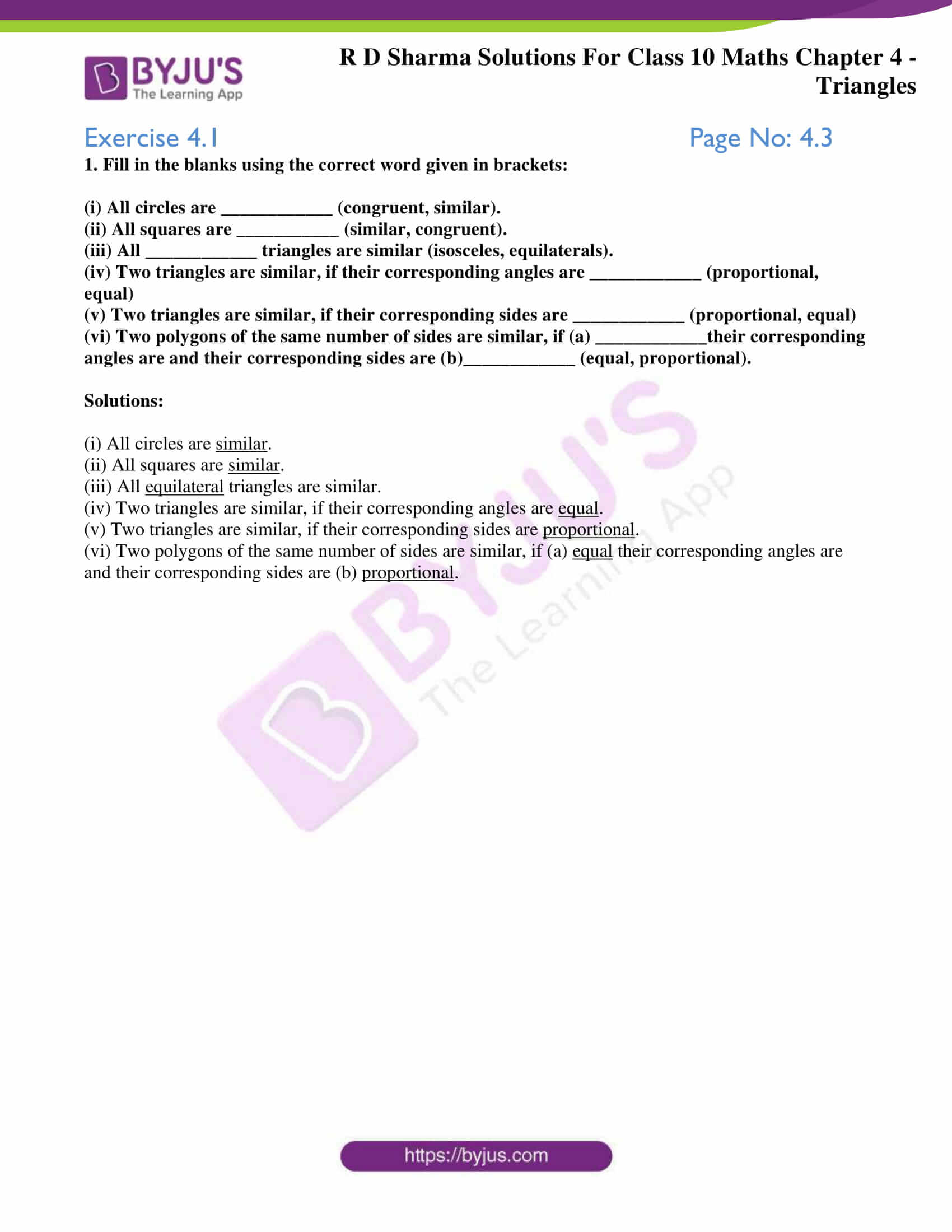# RD Sharma Solutions for Class 10 Maths Chapter 4 Triangles Exercise 4.1

Triangles chapter is one of the important chapters in Class 10 Mathematics. The first exercise gives a basic understanding of the concept of similarity and a small brief on similar polygons. Students can clear any conceptual doubts by referring to RD Sharma Solutions Class 10 which are prepared by subject experts at BYJU’S. Also, students can make use of the RD Sharma Solutions for Class 10 Maths Chapter 4 Triangles Exercise 4.1 PDF provided below.

## RD Sharma Solutions for Class 10 Maths Chapter 4 Triangles Exercise 4.1 Download PDF### Access RD Sharma Solutions for Class 10 Maths Chapter 4 Triangles Exercise 4.1

1. Fill in the blanks using the correct word given in brackets:

(i) All circles are ____________ (congruent, similar).

(ii) All squares are ___________ (similar, congruent).

(iii) All ____________ triangles are similar (isosceles, equilaterals).

(iv) Two triangles are similar, if their corresponding angles are ____________ (proportional, equal)

(v) Two triangles are similar, if their corresponding sides are ____________ (proportional, equal)

(vi) Two polygons of the same number of sides are similar, if (a) ____________their corresponding angles are and their corresponding sides are (b)____________ (equal, proportional).

Solutions:

(i) All circles are similar.

(ii) All squares are similar.

(iii) All equilateral triangles are similar.

(iv) Two triangles are similar, if their corresponding angles are equal.

(v) Two triangles are similar, if their corresponding sides are proportional.

(vi) Two polygons of the same number of sides are similar, if (a) equal their corresponding angles are and their corresponding sides are (b) proportional.